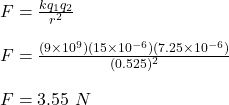## An object carries a +15.5 µC charge. It is 0.525 m from a -7.25 µC charge. What is the magnitude of the electric force on the object?

Question

An object carries a +15.5 µC charge. It is 0.525 m from a -7.25 µC charge. What is the magnitude of the electric force on the object?

in progress 0
4 months 2021-09-05T13:27:21+00:00 1 Answers 7 views 0

## Answers ( )

the force of attraction between the two charges is 3.55 N.

Explanation:

Given;

first charge carried by the object, q₁ = 15.5 µC

second charge carried by the q₂ = -7.25 µC

distance between the two charges, r = 0.525 m

The force of attraction between the two charges is calculated as;Therefore, the force of attraction between the two charges is 3.55 N.